Normal Math-Formulas

Introduction

Following you find a nomination of various mathematical formulas. They should be a reference and also be helpful, to close possibly knowledge gaps. Because without good math knowledge complex games and applications can't be realized.

Note: Instead of the used sign '^' please use the PureBasic function Pow() from the 'Math' library. 'PI' stands for the constant value "PI = 3.1415...". An example for realizing such a formula in PureBasic code: A = PI*r^2 will be A = PI*Pow(r,2)

Squarel = Side length
e = Corner measure (ment)
A = Surface
U = Extent

Surface: A = l^2

Extent: U = 4*l

Corner measure (ment): e = SQR(2)*l

Rhombus/Lozengel = Side length
b = Width
A = Surface
U = Extent

Surface: A = l*b

Extent: U = 4*l

Rectangle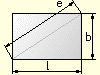l = Length
b = Width
e = Corner measure (ment)
A = Surface
U = Extent

Surface: A = l*b

Extent: U = 2*l+2*b

Corner measure (ment): e = SQR(l^2+b^2))

Rhomboid/Parallelogramm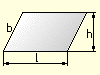l = Lengthens
h = Height
b = Width
A = Surface
U = Extent

Surface: A = l*h

Extent: U = 2*l+2*b

Trapezoida = Side 1
b = Side 2
c = Side 3
d = Side 4
lm = Middle length
h = Height
A = Surface
U = Extent

Surface: A = (a+c)/2*h

Extent: U = a+b+c+d

Middle Length: lm = (a+c)/2

Trianglea = Side 1
b = Side 2
c = Side 3
l = Side length
h = Height
A = Surface
U = Extent

Surface:
A = (l*h)/2
A = 1/4*SQR(U*(U-2*a)*(U-2*b)*(U-2*c))

Extent: U = a+b+c

Polygon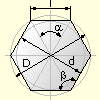l = Side length
d = Interior circle diameter
D = Periphery diameter
n = Number of corners
a = Central angle
ss = Corner angle
A = Surface
U = Extent

Interior circle diameter: d = SQR(D*D-l*l)

Periphery diameter: D = SQR(d*d+l*l)

Side length: l = D*SIN(180/n)

Central angle: a = 360/n

Corner angle: ss = 180-a

Surface: A = n*l*d/4

Extent: U = l*n

Circle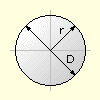D = Diameter
A = Surface
U = Extent

Surface:
A = PI*r^2
A = PI/4*d^2

Extent:
U = 2*PI*r
U = PI*d

Sector of a circle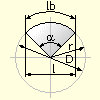D = Diameter
l = Sinew length
lb = Length of curve
a = Central angle
A = Surface
U = Extent

Sinew length: l = 2*r*SIN(a/2)

Length of curve: lb = PI*r*a/180

Surface:
A = PI*D^2*a/1440
A = lb*r/2

Extent: U = lb+D

Circle section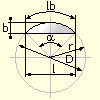D = Diameter
l = Sinew length
lb = Length of curve
a = Central angle
b = Width
A = Surface
U = Extent

Sinew Length:
l = 2*r*SIN(a/2)
l = 2*SQR(b*(2*r-b))

Length of curve: lb = PI*r*a/180

Width:
b = l/2*TAN(a/4)
b = r-SQR(r^2-l^2/4)

Radius: r = b/2+l^2/(8*b)

Surface:
A = PI*d^2*a/1440-(l*(r-b))/2
A = (lb*r-l*(r-b))/2

Extent: U = lb+l

Annulusd = Inside diameter
D = Outside diameter
dm = Middle diameter
b = Width
A = Surface

Middle diameter: dm = (D+d)/2

Surface:
A = PI*dm*b
A = PI/4*(D^2-d^2)

Memory consumption of a picture

a = Width of picture (in Pixel)
b = Height of picture (in Pixel)
bits = Colordepth of the picture (in Bits)

size = a * b * (bits / 8)

Distance between two points

(x1|y1) = XY coordinates of point No. 1
(x2|y2) = XY coordinates of point No. 2
a = Distance between the points

a = SQR((x1-x2)^2 + (y1-y2)^2)

"Mixing" of two values

a = 1st Number
b = 2nd Number
v = Relationship (0% - 100%)
e = Result

e = ((a*v)/100) + ((b*(100-v)) / 100)